Problem

# Frederick Schut and Peter VanBergeijk16 published an article in which they attempted to...

Frederick Schut and Peter VanBergeijk16 published an article in which they attempted to see if the pharmaceutical industry practiced international price discrimination by estimating a model of the prices of pharmaceuticals in a cross section of 32 countries. The authors felt that if price discrimination existed, then the coefficient of per capita income in a properly specified price equation would be strongly positive. The reason they felt that the coefficient of per capita income would measure price discrimination went as follows: the higher the ability to pay, the lower (in absolute value) the price elasticity of demand for pharmaceuticals and the higher the price a price discriminator could charge. In addition, the authors expected that prices would be higher if pharmaceutical patents were allowed and that prices would be lower if price controls existed, if competition was encouraged, or if the pharmaceutical market in a country was relatively large. Their estimates were (standard errors in parentheses):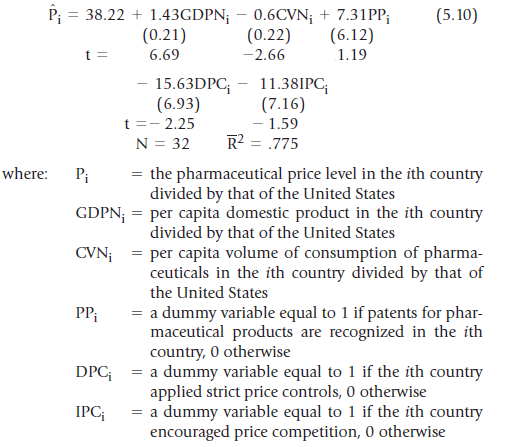where: Pi = the pharmaceutical price level in the ith country divided by that of the United States

GDPNi = per capita domestic product in the ith country divided by that of the United States

CVNi = per capita volume of consumption of pharmaceuticals in the ith country divided by that of the United States

PPi = a dummy variable equal to 1 if patents for pharmaceutical products are recognized in the ith country, 0 otherwise

DPCi = a dummy variable equal to 1 if the ith country applied strict price controls, 0 otherwise

IPCi = a dummy variable equal to 1 if the ith country encouraged price competition, 0 otherwise

a. Develop and test appropriate hypotheses concerning the regression coefficients using the t-test at the 5-percent level. b. Set up 90-percent confidence intervals for each of the estimated slope coefficients. c. Do you think Schut and VanBergeijk concluded that international price discrimination exists? Why or why not? d. How would the estimated results have differed if the authors had not divided each country’s prices, per capita income, and per capita pharmaceutical consumption by that of the United States? Explain your answer. e. Reproduce their regression results by using the EViews computer program (datafile DRUGS5) or your own computer program and the data from Table 5.1.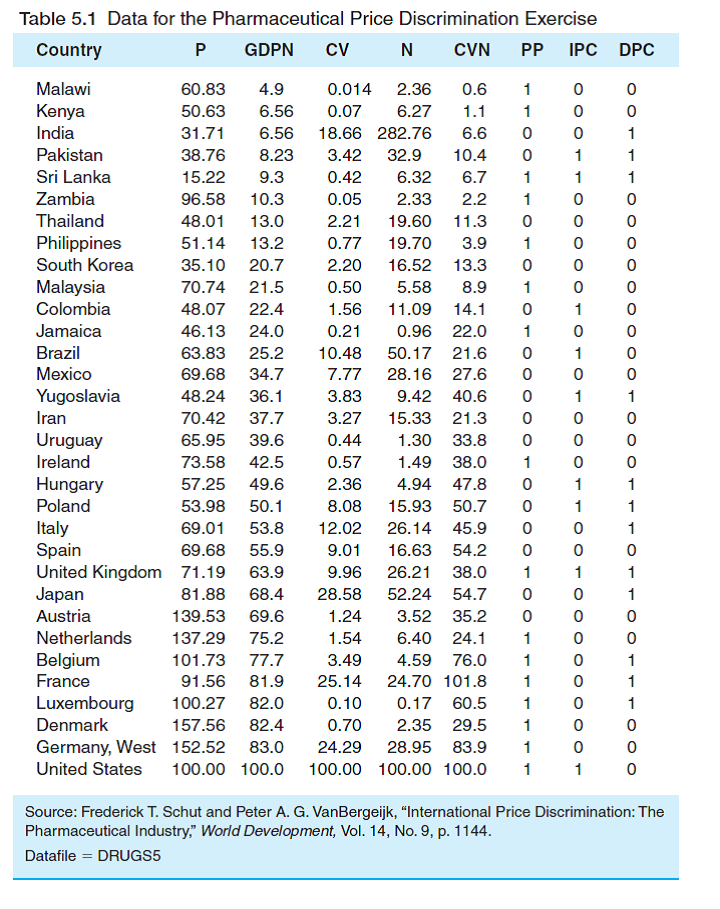#### Step-by-Step Solution

Solution 1

Equation (1) examines the price discrimination in the pharmaceutical industry for 32 countries.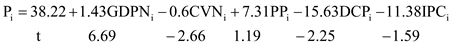…… (1)

where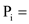the pharmaceutical price level in the ith country divided by that of the United States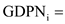per capita domestic product in the ith country divided by that of the United States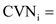per capita volume of consumption of pharmaceutical products in the ith country divided by that of the United States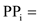a dummy variable equal to 1 if patents for pharmaceutical products are recognized in the ith country and 0 otherwise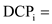a dummy variable equal to 1 if the ith country applied strict price controls and 0 otherwise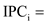a dummy variable equal to 1 if the ith country encouraged price competition and 0 otherwise

a.

Framing and testing of hypothesis:

Framing hypothesis for coefficient of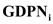:

The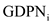variable is expected to have a positive sign; hence, the null and alternative hypotheses are as follows: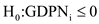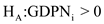For coefficient of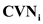:

The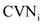variable is expected to have a negative sign; hence, the null and alternative hypotheses are as follows: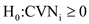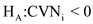For coefficient of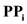The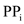variable is expected to have a positive sign; hence, the null and alternative hypotheses are as follows: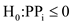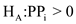For coefficient of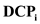The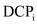variable is expected to have a negative sign; hence, the null and alternative hypotheses are as follows: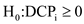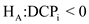For coefficient of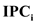The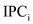variable is expected to have a negative sign; hence, the null and alternative hypotheses are as follows: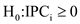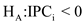Testing of Hypothesis:

Hypothesis testing will be done to verify whether the estimated coefficients are statistically significant or not.

To reject the null hypothesis, the computed t value of the kth coefficient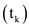should be greater than the critical t value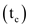.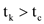The calculated t value of the coefficient of variables,,,, andis given in Equation (1), and it is represented as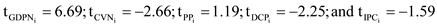, respectively.

To obtain the critical t value, refer to the t distribution table, where the respective column (5-percent level of significance) and row (26 degrees of freedom) intersect each other.where

N = the number of observations

K = the number of coefficients included in the equation, including

Constant.

The critical value at the five-percent level of significance is 1.706 with the degrees of freedom of 26.

The sign of t value carries the sign of coefficients; thus, the sign will not be taken into account while comparing calculated and critical t values.

Hypothesis testing : coefficient ofThe calculated t value is greater than the critical t value.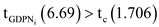Hence, the null hypothesis is rejected; the estimated coefficientis statistically significant at the 5-percent level of significance with an expected sign mentioned in the alternative hypothesis.

Hypothesis testing : coefficient ofThe calculated t value is greater than the critical t value.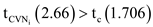Hence, the null hypothesis is rejected; the estimated coefficientis statistically significant at the 5-percent level of significance with an expected sign mentioned in the alternative hypothesis.

Hypothesis testing : coefficient ofThe calculated t value is less than the critical t value.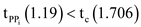Hence, we fail to reject the hypothesis; the estimated coefficientis statistically insignificant at the 5-percent level.

Hypothesis testing : coefficient ofThe calculated t value is greater than the critical t value.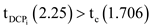Hence, the null hypothesis is rejected; the estimated coefficientis statistically significant at the 5-percent level of significance with an expected sign mentioned in the alternative hypothesis.

Hypothesis testing : coefficient ofThe calculated t value is less than the critical t value.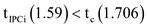Hence, we fail to reject the null hypothesis; the estimated coefficientis statistically insignificant at the 5-percent level.

b.

90 percent confidence interval for all the estimated slope coefficients:

In Equation (1), the size of N is 32, and the number of parameters K is 6; hence, the degrees of freedom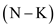is 26.

The 90% confidence interval for 26 degrees of freedom is 1.315.

In a one-tail test, the null hypothesis will not be rejected, if the calculated t value is less than 1.315.

Confidence interval for coefficient of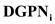:

The standard formula to calculate confidence interval for an estimated coefficient is as follows.

Confidence interval =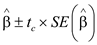…… (2)

Where,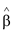= Estimated coefficient of respective variable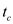= Critical value

SE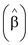= Standard error of estimated coefficient

Hence, 90 percent confidence interval for coefficient ofisTherefore, the 90 percent confidence interval ofshows that the true coefficient will fall within the range of 1.70615 to 1.15385 90percent times.

Confidence interval for coefficient of:

Substitute the respective values ofHence the true coefficient value will fall in between the range of 0.8893 to 0.3107

Confidence interval for coefficient ofSubstitute the respective values ofHence, the true coefficient value will fall in between 15.3578 and -0.7378

Confidence interval for coefficient of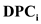Substitute the respective values ofHence, the true coefficient value will fall in between – 6.51705 to -24.74295

Confidence interval for coefficient ofSubstitute the values ofHence, the true coefficient value will fall in between -1.9646 to -20.7954

c.

Price discrimination exists in the pharmaceutical industry:

Yes, because the coefficient of the variable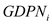in Equation (1) is positive and highly significant at the 5-percent level, so the study concluded that international price discrimination exist in the pharmaceutical industry.

d.

Change in measurement of the variable will affect the estimated result:

The change in measurement of the variable will affect the estimated result.

If each country’s prices, per capita income, and per capita pharmaceutical consumption are not divided by those of the United States, then the value of the respective variables would have changed. This in turn will lead to a change in the estimated results because the values of the coefficient are determined by the values of the variable.

Hence, the estimated results will differ.

e. Estimated result of Equations (1) and (2) are same

The following equation estimates the price discrimination in the pharmaceutical industry for 32 countries: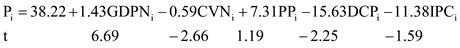……(2)

Equation (2) shows the estimated results obtained from the Stata software.

The estimated results obtained from the Stata software and the results shown in Equation (1) are the same.

Table (1) shows the actual output obtained from the Stata software.

Table (1)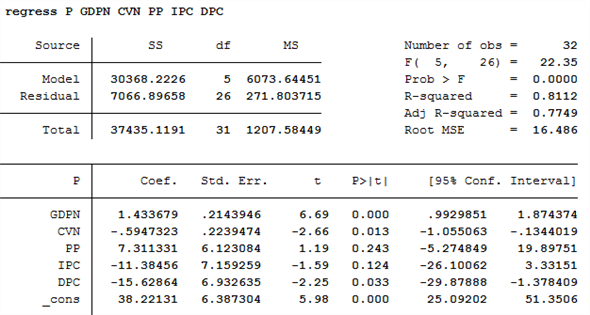Add your Solution
Textbook Solutions and Answers Search
Solutions For Problems in Chapter 5